# Beyond Mapping III

Topic 2: Spatial Interpolation Procedures and Assessment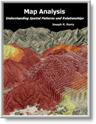Map Analysis book with companion CD-ROM for hands-on exercises

The Average Is Hardly Anywhere  discusses the difference between spatial and non-spatial data distributions

Under the Hood of Spatial Interpolation  investigates the basic concepts in IDW and Krig interpolation procedures

describes the "Residual Analysis" procedure for assessing interpolation performance
discusses a technique for generating a "shadow map" of error
describes how normalized maps of error can be used to visualize the differences in error surfaces

Note: The processing and figures discussed in this topic were derived using MapCalcTM software.  See www.innovativegis.com to download a free MapCalc Learner version with tutorial materials for classroom and self-learning map analysis concepts and procedures.

______________________________

The Average is Hardly Anywhere

(GeoWorld May, 2006)

Remember your first encounter with statistics?  In the days of old before politically correct examples, you might have calculated the average weight of the students in the class by adding up all of the students’ weights and then dividing by the number of students.  The average weight gives you an estimate of how heavy a “typical student” is and the standard deviation tells you how typical that typical is.

Now imagine your old classroom with the bulky jocks in the back, the diminutive bookworms in front, and the rest caught in between.  Two things should come to mind—1) not all of the students had the same typical weight (some were heavier and some were lighter) and 2) the differences from the typical weight might have followed a geographic pattern (heavy in the back, light in the front).  The second point forms the foundation of surface modeling that “maps the variation in geographic data sets.”

Figure 1 illustrates the spatial and non-spatial character of a set of animal activity data.  The right side of the figure lists the number of sightings at sixteen locations for two 24-hour periods (P1 in June; P2 in August).  Note the varying levels of activity— 0 to 42 for P1 and 0 to 87 for P2 that can be reduced to their average values of 19 and 23, respectively.  A wildlife manager might ponder these findings, and then determine whether the implied typical activity is too little, too much or just right for a particular management action.

Figure 1.  Comparison of the spatial distributions of field samples (floating balls) and their arithmetic average (horizontal plane).

But the average does not address the variation in the data set— that's the role of the standard deviation.  As a general rule (termed the Coefficient of Variation) “…if the standard deviation is relatively large compared to the arithmetic average, the average cannot be used to make decisions” as there is too much unexplained variation in the data (i.e., the computed average isn't very typical).

Possibly some of the variation in animal activity forms a pattern in geographic space that might help extend the explanation.  That is where the left side of figure 1 comes into play with a three-dimensional plot used to show the geographic location (X, Y) and the measured activity levels (Z).  I'll bet your eye is “mapping the variation in the data” as high activity in the Northeast, low in the Northwest and moderate elsewhere.

The thick line in the plot outlines a horizontal plane at 23 (arithmetic average) that spatially characterizes the typical animal activity as uniformly distributed in geographic space (horizontal plane).  But your eye tells you that guessing 23 around Sample #15 with a measured value of 87, is likely an understatement.  Similarly, a guess of 23 around Sample #4 with a value of 0 is likely an overstatement.  That is what the relatively large standard deviation indicated— guess 23 but expect to be way off (+ 26) a lot of the time.

The non-spatial procedure, however, doesn’t provide a hint as to where the average might be guessing too low and where it might be guessing high.  That's the main difference between traditional statistics and spatial statistics— traditional statistics characterizes the central tendency (average) of data in numeric space; spatial statistics seeks to map the variation (standard deviation) of data in geographic space.

Figure 2 illustrates Map Generalization as an approach to mapping the spatial trend in the data using polynomial surface fitting.  The average of 23 is depicted as a horizontal plane that does its best to balance half of the balls above it and half below it by minimizing the set of squared deviations from the plane to each floating ball (similar to curve-fitting regression techniques in traditional statistics).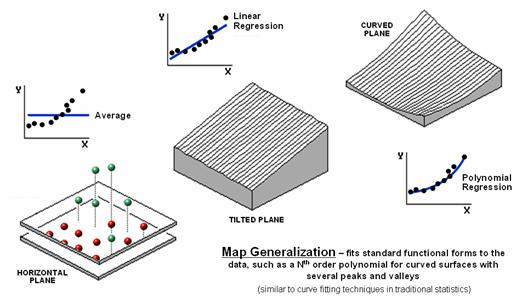Figure 2.  Map Generalization can be used to approximate geographic trends.

Now relax the assumption that the plane has to remain horizontal.  Tilt it every-which-way until it better fits the floating balls (Tilted Plane).  Or even better, the assumption of a flat plane can be relaxed and the surface can curve to better fit the ups and downs of the data points.  The right portion of the figure fits a 2nd degree polynomial (Curved Plane).

Figure 3 shows an entirely different approach to fitting a surface to the data by using the Spatial Interpolation technique of iterative smoothing.  Imagine replacing the floating balls with columns of modeler's clay rising to the same height as each ball.  In effect this is a blocky first-order estimate of the animal activity throughout the project area derived by simply assigning the closest field sample to each map location.

Now imagine whacking away some of the clay at the top of the columns and filling-in at the bottom.  In the example, a 3x3 averaging window was moved around the matrix of values to systematically smooth the blocky surface.  When the window is centered over one of the sharp boundaries, it has a mixture of small and larger map values, resulting in an average somewhere in between... a localized whack off the top and a fill-in at the bottom.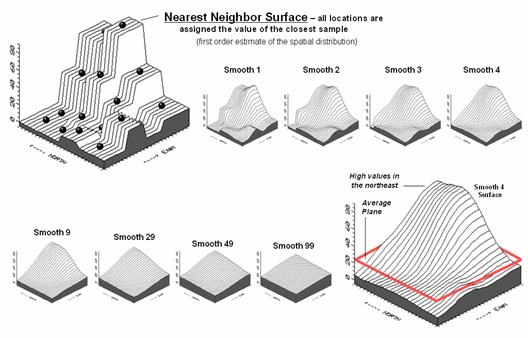Figure 3.  Iteratively Smoothed approximations of localized geographic distribution.

The series of plots in the figure show repeated passes of the smoothing window from once through ninety-nine times.  Like erosion, the mountains (high animal activity) are pulled down and the valleys (low animal activity) are pulled up.  According to theory, the process eventually approximates a horizontal plane floating at the arithmetic average.

The bottom line is that field collected data with geographic coordinates holds a lot more information than simply a reduction to a typical value.  Next month’s column will investigate other more powerful methods for estimating the spatial, as well as numerical distribution inherent in mapped data.

(GeoWorld June, 2006)

Last month's article described how field collected data (discrete points) can be used to generate a map (continuous surface) of the data’s spatial patterns.  The derived surface extends the familiar concept of central tendency to a map of the geographic distribution of the data.  Whereas traditional statistics identifies the typical value in a data set, surface modeling identifies "where" you might expect to find the typical and not so typical responses.

The Iterative Smoothing approach described last time is a simple data-driven procedure.  However, all of the interpolation techniques share a similar approach that generates estimates of a mapped variable based on the data values within the vicinity of each map location.  In effect, this establishes a "roving window" that moves throughout an area summarizing the field samples it encounters within its reach.  The summary estimate is assigned to the center of the window, and then it moves on.  The extents of the window (both size and shape) influence the result, regardless of the summary technique.  In general, a large window capturing numerous values tends to smooth the data.  A smaller window tends to result in a rougher surface.

Three factors affect the window's configuration— its reach, number of samples, and balancing.  The reach, or search radius, sets a limit on how far the computer will go in collecting data values.  The number of samples establishes how many data values will be used.  The balancing of the data attempts to eliminate directional bias by insuring that values are selected from all directions around the window's center.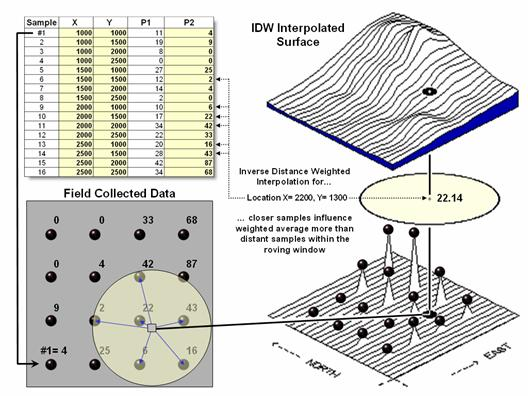Figure 1. A roving window is used to identify and summarize nearby sample values.

Once a window is established, the summary technique comes into play.  The Inverse Distance Weighted (IDW) technique is easy to conceptualize (see figure 1).  It estimates a value for a location as the weighted-average of the nearby data values within the roving window.  The average is weighted so the influence of the surrounding values decrease with increasing distance from the location being estimated.

Figure 2 shows the IDW calculations for the location identified in figure 1.  The Pythagorean Theorem is used to calculate the geographic distance between the location and the six sample values in the window.  A weight for each sample is determined as 1/D2, then the weighted average of the samples is calculated and assigned to the location.  The process is repeated for each location in the project area.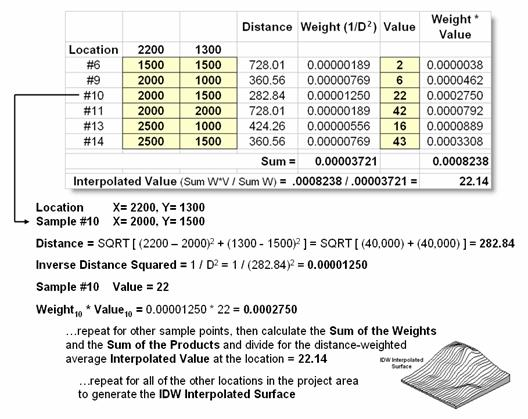Figure 2. IDW calculations use simple geographic distance to weight the average of samples within a roving window.

Because IDW is a static averaging method, the estimated values can never exceed the range of values in the original sample data.  Also, it tends to "pull-down peaks and pull-up valleys" in the data.  Inverse distance is best suited for data sets where the samples are fairly independent of their surrounding locations (i.e., no strong regional trend).

Another approach, Kriging (KRIG) uses regional variable theory based on an underlying variogram.  That's techy-speak implying that there is a lot of math behind the approach.  In effect, the technique develops custom weights based on trends in the sample data.  The moving average weights that define the data trends can result in estimated values that exceed the field data's range of values.  Also, there can be unexpected results in large areas without data values.  The technique is most appropriate for fairly dense, systematically sampled data exhibiting discernable regional trends.

The center portion figure 3 illustrates how the Krig weights are derived.  The distances between each sample point and all of the other sample points are calculated.  At the same time, the differences in the sample values are recorded.  Common sense suggests that “nearby things are more alike than distant things” and a plot of Distance versus Difference often looks something like the variogram in the center of the figure.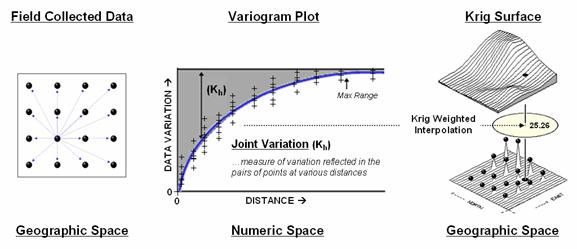Figure 3. A variogram plot depicts the relationship between distance and similarity between sample values.

The origin of the plot at 0,0 is a unique case.   The distance between samples is zero; therefore, there shouldn’t be any dissimilarity (data variation = zero) as the location is exactly the same as itself.  As the distance between points increase, subsets of the data are scrutinized for their dependency.  The shaded portion in the idealized plot shows a rapid deterioration of the spatial dependency among the sample points.  The maximum range (Max Range) position identifies the distance between points beyond which the data values are considered independent.  This suggests that using data values beyond this distance for interpolation isn’t useful.

An equation is fit to the Distance-Difference data and used to determine the weights and reach used in averaging the sample values within the roving window.  Whereas IDW uses a fixed geometric function, Krig derives the weights by investigating the spatial dependency in the sample data (see Author’s Note).  Keep in mind that most analytical GIS systems have a dozen or more spatial interpolation techniques— but they all share the common basics of a window configuration and a summary procedure.

______________________________

Author’s Note:  Let me apologize in advance for such a terse treatment of a complex subject.  Topic 8, Investigating Spatial Dependency of the online book Map Analysis ( www.innovativegis.com/basis/) discusses in greater depth the various measures of spatial autocorrelation, their interpretation and use in interpolation.

(GeoWorld, February 1997, pg. 34)

Several previous Beyond Mapping articles have discussed the basic considerations in generating maps from point data.  Recall that both sampling design and interpolation technique greatly affect the results when converting discrete point samples (e.g., soil samples) to continuous map surfaces (e.g., map of phosphorous levels throughout a field).  The only thing for certain is that there isn’t a single best procedure that covers all situations.  Some rules of thumb and general considerations have been discussed, but the only good way to determine the “best” map is to empirically verify the results.  This involves generating several maps under different sampling/interpolation procedures, then testing the results against a set of known measurements, aptly termed a “test set.”  As in horseshoes and boche ball, the closest one wins.

The accompanying table show large differences among three interpolated surfaces generated from the same set of point sampled data, termed the “interpolation set.”  The algorithmic nuances and relative advantages and disadvantages of the Inverse Distance, Kriging and Minimum Curvature techniques where discussed in GW Vol 7, No 1-3.  What should spring to mind at this point is “OK, they’re different, so how can I tell which is best?”  An empirical verification technique, termed residual analysis, summarizes the differences between interpolation estimates and actual measurements for each test location.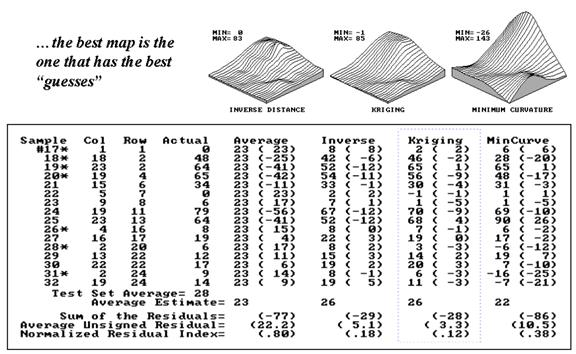Figure 1.  Residual table identifying actual and estimated values.

The table in figure 1 contains a test set of sixteen random samples (#17-32) used to evaluate three maps.  The “Actual” column lists the measured values for the test locations identified by “Col, Row” coordinates.  The difference between these values and those predicted by the three interpolation techniques form the residuals shown in parentheses.  The “Average” column compares the whole field arithmetic mean of 23 (guess 23 everywhere) for each test location.

For example, the first sample (#17) guessed 23 but was actually 0, therefore it’s off by 23.  Note the sign of the residual indicates whether the guess was too high (positive) or too low (negative).  If it was a perfect data world, you would expect the Sum of the Residuals to be 0 (just as many high as low misses— balanced boo-boo).  However, in this case there is a net residual of -77 indicating a strong bias to under estimate.  The Average Unsigned Residual is simply the average magnitude of mistakes, regardless whether they’re over or under.  For the “Average” estimate expect to be off by about 22.2 each time you guess.  The Normalized Residual Index is simply the ratio of the Average Unsigned Residual to the Test Set Average.  An index of .80 (22.2/28) indicates that the “Average” technique isn’t very good at spatial estimates.

Now take a look at the residual analysis for the three interpolation techniques.  All three are considerably better than the whole field average (Normalized Residual Indices of .18, .12 and .38, respectively, compared to the whopping .80 for the simple whole field average).  The “Kriging” technique is best, having a 3.3 Average Unsigned Residual with a tendency to under estimate (a Sum of the Residuals of -28).  The “Inverse” technique is a close second with an Average Unsigned Residual of 5.1 and a nearly identical under estimate bias of -29.  The “MinCurve” technique indices indicate that it is a distant third, but still much better than using the whole field average for spatial estimates.

However, we haven’t considered other potential affects.  The asterisks identify test measurements outside the set of samples used for generating the map surfaces.  Estimating these values is termed spatial extrapolation and some techniques are better than others.  I wonder if there are significant differences among the three techniques in their spatial interpolation and extrapolation estimates (get out your calculator)?  Would you expect the conclusions to change if the “test” and “interpolation” data samples were swapped and another residual analysis performed?  What if different sampling designs were tried.  What about different interpolation parameters (window reach, number of samples and balancing)?  At least residual analysis gives us a place to start looking for answers.

Move Beyond a Map Full of Errors

(GeoWorld, March 1997, pg. 28)

Last month’s article described a procedure for checking the reliability of maps generated from field samples, such as a map of phosphorous levels derived from a set of soil samples.  The data could have as easily been lead concentrations in an aquifer from a set of sample wells, or any other variable that forms a gradient in geographic space for that matter.  The evaluation procedure suggested holding back some of the samples as a test set to “empirically verify” the estimated value at each location.  Keep in mind, if you don’t attempt to verify, then you are simply accepting the derived map as a perfect rendering of reality (and blind faith isn’t a particularly a good basis for visual analysis of mapped data).  The difference between a mapped “guess” (interpolated estimate) and “what is” (actual measurement) is termed a residual.

A table of all the residuals for a test set is summarized to determine the overall accuracy of an interpolated map.  The residual analysis described last time determined that the Kriging interpolation procedure had the closest predictions to those of the example test set.  Its predictions, on average, were only 3.3 units off, whereas the other techniques of Inverse Distance and Minimum Curvature were considerably less accurate (5.1 and 10.5, respectively).  The use of an arithmetic average for spatial prediction was by far the worst with an average boo-boo of 22.2 for each guess.  The residual table might have identified the relative performance of the various techniques, but it failed to identify where a map is likely predicting too high or too low— that’s the role of a residual map.

The table in Figure 1 shows the actual and estimated values from Kriging for each test location, with their residuals shown in parentheses. The posted values on the 3-D surface locate the positioning of the residual values.

For example, the value 1 posted in the northeast corner identifies the residual for test sample #19; the 4 to its right identifies the residual for test sample #25 along the eastern edge of the field.  The map of the residuals was derived by spatially interpolating the set of residuals.  The 3-D rendering shows the residual surface, while the 2-D map shows 1-unit contours of error from -9 (under guessed by nine) to 4 (over guessed by four).  The thick, dark lines in both plots locates the zero residual contour— locations believed to be right on target.  The darker tones identify areas of overestimates, while the lighter tones identify areas of underestimates.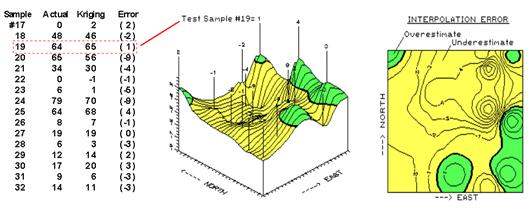Figure 1.  Map of errors from the Kriging interpolation technique.

As explained last time, the sum of the residuals (-28) indicates a overall tendency of the Kriging technique to underestimate the data used in this example.  The predominance of lighter tones in the residual map spatially supports this overall conclusion.  While the underestimated area occurs throughout the center of the field, it appears that Kriging’s overestimates occur along the right (east), bottom (south) and the extreme upper left (northwest) and upper right (northeast) corners.

The upper right portion (northeast) of the error map is particularly interesting.  The two -9 “holes” for test samples #20 and #24 quickly rise to the 1 and 4 “peaks” for samples #19 and #25.  Such wide disparities within close proximity of each other (steep slopes in 3-D and tight contour lines in 2-D) indicate areas of wildly differing interpolation performance.  It’s possible that something interesting is going on in the northeast and we ought to explore a bit further.  Take a look at the data in the table.  The four points represent areas with the highest responses (65, 56, 70 and 78).  Come to think of it, a residual of 1 on an estimate of 64 for sample #19 really isn’t off by much (only 1.5%).  And its underestimated neighbor’s of 9 on 65 (sample #20) isn’t too bad either (14%).

Maybe a map of “normalized” residuals might display the distribution of error in a more useful form.  What do you think?  Are you willing to “go the distance” with the numbers?  Or simply willing to “click” on an icon in your GIS, sit back, and blindly enjoy the colorful renderings of automated mapping.  At very least, the past few articles on sampling and assessing interpolation results should have taken your thoughts “beyond pretty pictures” toward “pretty useful mapped data”… remember, all GIS maps are organized sets of numbers and those numbers might be trying to tell you something.
_____________________________
Author's Note:  Residual Analysis and Mapping was briefly presented in discussions about map uncertainty (GW Volume 7, Numbers 7-10).

Comparing Map Errors

(GeoWorld, April 1997, pg. 26)

The previous article challenged you to consider what advantage a normalized map of residuals might have.  Recall that an un-normalized map was generated from the boo-boos (more formally termed residuals) uncovered by comparing interpolated estimates with a test set of known measurements.  Numerical summaries of the residuals provided insight into the overall interpolation performance, whereas the map of residuals showed where the guesses were likely high and where they were likely low.

The map on the left side of figure 1 is the “plain vanilla” version of the error map discussed in the previous article.  The one on the right is the normalized version.  See any differences or similarities?  At first glance, the sets of lines seem to form radically different patterns.  A closer look reveals that the patterns of the darker tones are identical.  So what gives?

First of all, let’s consider how the residuals were normalized.  The arithmetic mean of the test set (28) was used as the common reference.  For example, test location #17 estimated 2 while its actual value was 0, resulting in an overestimate of 2 (2-0= 2).  This simple residual is translated into a normalized value of 7.1 by computing (0-2)/28)*100= 7.1, a signed (+ or -) percentage of the “typical” test value.  Similar calculations for the remaining residuals brings the entire test set in line with its typical, then a residual map is generated.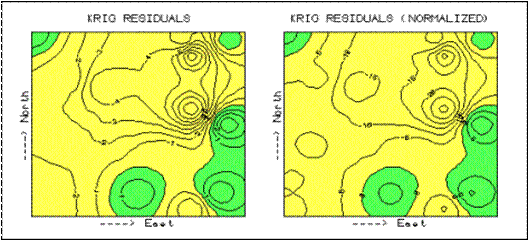Figure 1.  Comparison of error maps (2-D) using Absolute and Normalized Kriging residual values.

Now let’s turn our attention back to the maps.  As the techy-types among you guessed, the spatial pattern of interpolation error is not effected by normalization (nor is its numerical distribution)— all normalizing did was “linearly” re-scale the map surface.  The differences you detect in the line patterns are simply artifacts of different horizontal “slices” through the two related map surfaces.  Whereas a 5% contour interval is used in the normalized version, a contour interval of 1 is used in the absolute version.  The common “zero contour” (break between the two tones) in both maps have an identical pattern, as would be the case for any common slice (relative contour step).

Ok… but if normalizing doesn’t change a map surface, why would anyone go to all the extra effort?  Because normalizing provides the consistent referencing and scaling needed for comparison among different data sets.  You can’t just take a couple of maps, plop them on a light table, and start making comparative comments.  Everyone knows you have to adjust the map scales so they will precisely overlay (spatial registration).  In an analogous manner, you have to adjust their “thematic” scales as well.  That’s what normalization does.

Now visually compare magnitude and pattern of error between the Kriging and the Average surfaces in figure 2.  A horizontal plane aligning at zero on the Z-axis would indicate a “perfect” residual surface (all estimates were exactly the same as their corresponding test set measurements).  The Kriging plot on the left is relatively close to this ideal which confirms that the technique is pretty good at spatially predicting the sampled variable.  The surface on the right identifying the “whole field” Average technique shows a much larger magnitude of error (surface deflection from Z= 0).  Now note the patterns formed by the light and dark blobs on both map surfaces.  The Kriging overestimates (dark areas) are less pervasive and scattered along the edges of the field.  The Average overestimates occur as a single large blob in the southwestern half of the field.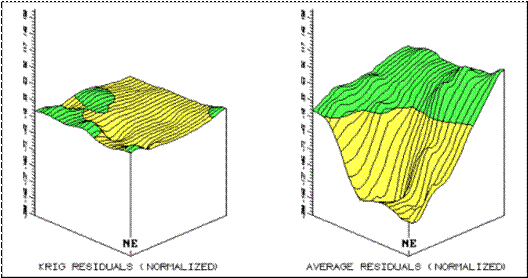Figure 2. Comparison of Residual Map Surfaces (3-D) Using Residual Values Derived by Kriging, and Average Interpolation Techniques.

What do you think you would get if you were to calculate the volumes contained within the light and dark regions?  Would their volumetric difference have anything to do with their Average Unsigned Residual values in the residual table discussed a couple of articles ago?  What relationship does the Normalized Residual Index have with the residual surfaces?  …Bah!  This map-ematical side of GIS really muddles its comfortable cartographic side— bring on the colorful maps at megahertz speed and damn the details.
_____________________________